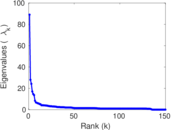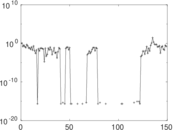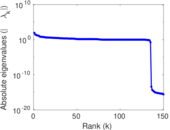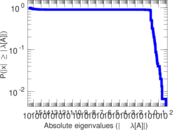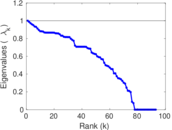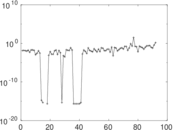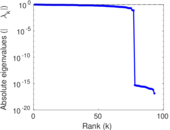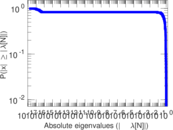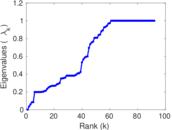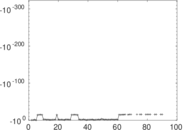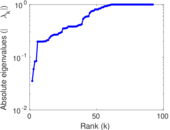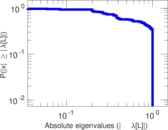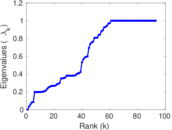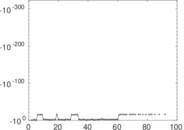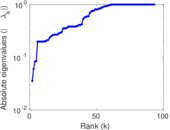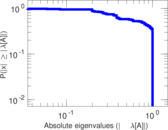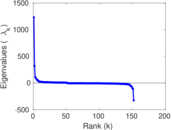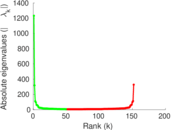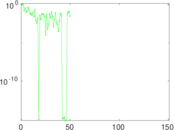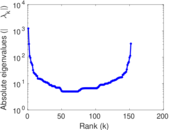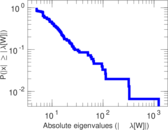# Wikibooks edits (li)

This is the bipartite edit network of the Limburgish Wikibooks. It contains users and pages from the Limburgish Wikibooks, connected by edit events. Each edge represents an edit. The dataset includes the timestamp of each edit.

 Code `bli` Internal name `edit-liwikibooks` Name Wikibooks edits (li) Data source http://dumps.wikimedia.org/ AvailabilityDataset is available for download Consistency checkDataset passed all tests Category Authorship network Dataset timestamp 2017-10-20 Node meaning User, article Edge meaning Edit Network formatBipartite, undirected Edge typeUnweighted, multiple edges Temporal dataEdges are annotated with timestamps

## Statistics

 Size n = 1,229 Left size n1 = 152 Right size n2 = 1,077 Volume m = 2,321 Unique edge count m̿ = 1,617 Wedge count s = 361,287 Claw count z = 86,368,550 Cross count x = 16,624,056,950 Square count q = 42,326 4-Tour count T4 = 1,790,194 Maximum degree dmax = 1,277 Maximum left degree d1max = 1,277 Maximum right degree d2max = 61 Average degree d = 3.777 05 Average left degree d1 = 15.269 7 Average right degree d2 = 2.155 06 Fill p = 0.009 877 58 Average edge multiplicity m̃ = 1.435 37 Size of LCC N = 1,086 Diameter δ = 10 50-Percentile effective diameter δ0.5 = 1.840 93 90-Percentile effective diameter δ0.9 = 3.871 54 Median distance δM = 2 Mean distance δm = 2.822 48 Gini coefficient G = 0.703 139 Balanced inequality ratio P = 0.216 286 Left balanced inequality ratio P1 = 0.121 930 Right balanced inequality ratio P2 = 0.325 291 Relative edge distribution entropy Her = 0.739 685 Power law exponent γ = 4.077 28 Tail power law exponent γt = 2.401 00 Tail power law exponent with p γ3 = 2.401 00 p-value p = 0.000 00 Left tail power law exponent with p γ3,1 = 1.921 00 Left p-value p1 = 0.090 000 0 Right tail power law exponent with p γ3,2 = 2.531 00 Right p-value p2 = 0.000 00 Degree assortativity ρ = −0.288 545 Degree assortativity p-value pρ = 2.234 19 × 10−32 Spectral norm α = 89.034 3 Algebraic connectivity a = 0.035 406 4 Spectral separation |λ1[A] / λ2[A]| = 3.176 38 Controllability C = 958 Relative controllability Cr = 0.780 130

## Plots

### Fruchterman–Reingold graph drawing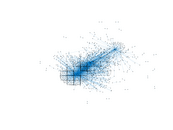### Degree distribution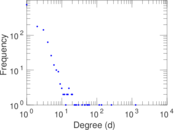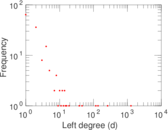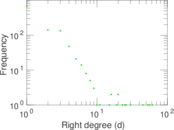### Cumulative degree distribution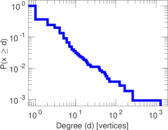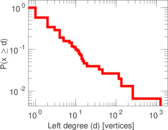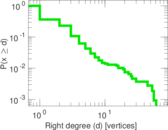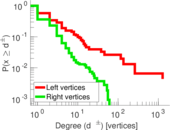### Lorenz curve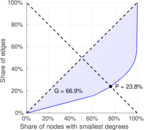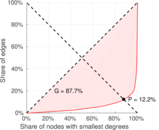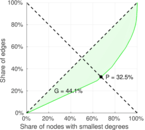### Spectral distribution of the adjacency matrix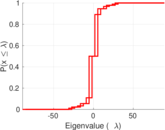### Spectral distribution of the normalized adjacency matrix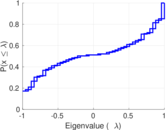### Spectral distribution of the Laplacian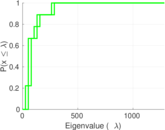### Spectral graph drawing based on the adjacency matrix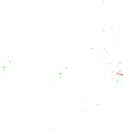### Spectral graph drawing based on the Laplacian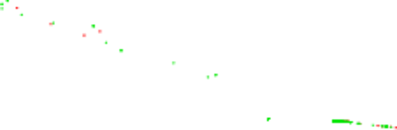### Spectral graph drawing based on the normalized adjacency matrix### Degree assortativity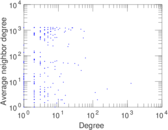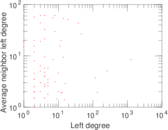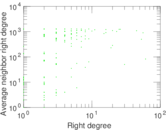### Zipf plot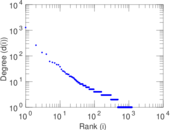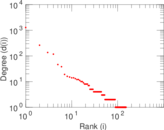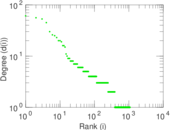### Hop distribution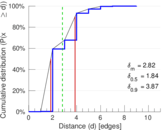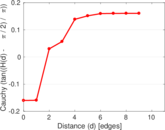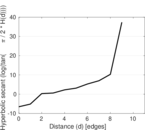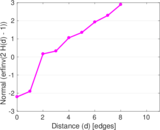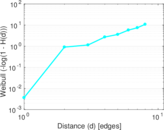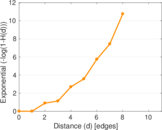### Double Laplacian graph drawing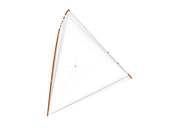### Delaunay graph drawing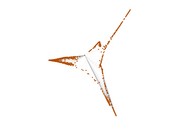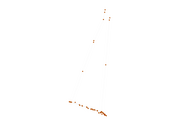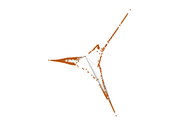### Edge weight/multiplicity distribution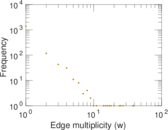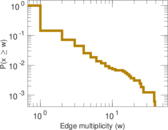### Temporal distribution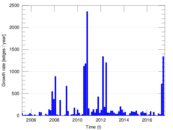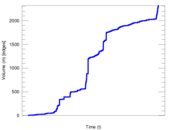### Temporal hop distribution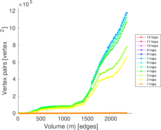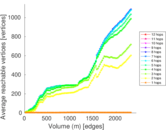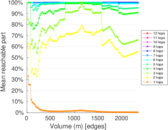### Diameter/density evolution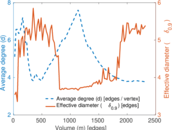### Matrix decompositions plots